## Uniform circular motion

9-29-99

Sections 5.1 - 5.2

### Uniform circular motion

When an object is experiencing uniform circular motion, it is traveling in a circular path at a constant speed. If r is the radius of the path, and we define the period, T, as the time it takes to make a complete circle, then the speed is given by the circumference over the period. A similar equation relates the magnitude of the acceleration to the speed: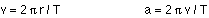These two equations can be combined to give the equation:This is known as the centripetal acceleration; v2 / r is the special form the acceleration takes when we're dealing with objects experiencing uniform circular motion.

### A warning about the term "centripetal force"

In circular motion many people use the term centripetal force, and say that the centripetal force is given by: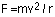I personally think that "centripetal force" is misleading, and I will use the phrase centripetal acceleration rather than centripetal force whenever possible. Centripetal force is a misleading term because, unlike the other forces we've dealt with like tension, the gravitational force, the normal force, and the force of friction, the centripetal force should not appear on a free-body diagram. You do NOT put a centripetal force on a free-body diagram for the same reason that ma does not appear on a free body diagram; F = ma is the net force, and the net force happens to have the special formwhen we're dealing with uniform circular motion.

The centripetal force is not something that mysteriously appears whenever an object is traveling in a circle; it is simply the special form of the net force.

### Newton's second law for uniform circular motion

Whenever an object experiences uniform circular motion there will always be a net force acting on the object pointing towards the center of the circular path. This net force has the special form, and because it points in to the center of the circle, at right angles to the velocity, the force will change the direction of the velocity but not the magnitude.

It's useful to look at some examples to see how we deal with situations involving uniform circular motion.

Example 1 - Twirling an object tied to a rope in a horizontal circle. (Note that the object travels in a horizontal circle, but the rope itself is not horizontal). If the tension in the rope is 100 N, the object's mass is 3.7 kg, and the rope is 1.4 m long, what is the angle of the rope with respect to the horizontal, and what is the speed of the object?

As always, the place to start is with a free-body diagram, which just has two forces, the tension and the weight. It's simplest to choose a coordinate system that is horizontal and vertical, because the centripetal acceleration will be horizontal, and there is no vertical acceleration.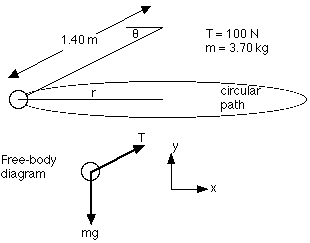The tension, T, gets split into horizontal and vertical components. We don't know the angle, but that's OK because we can solve for it. Adding forces in the y direction gives: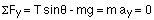This can be solved to get the angle: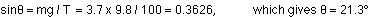In the x direction there's just the one force, the horizontal component of the tension, which we'll set equal to the mass times the centripetal acceleration:We know mass and tension and the angle, but we have to be careful with r, because it is not simply the length of the rope. It is the horizontal component of the 1.4 m (let's call this L, for length), so there's a factor of the cosine coming in to the r as well.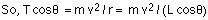Rearranging this to solve for the speed gives: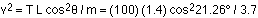which gives a speed of v = 5.73 m/s.

Example 2 - Identical objects on a turntable, different distances from the center. Let's not worry about doing a full analysis with numbers; instead, let's draw the free-body diagram, and then see if we can understand why the outer objects get thrown off the turntable at a lower rotational speed than objects closer to the center.

In this case, the free-body diagram has three forces, the force of gravity, the normal force, and a frictional force. The friction here is static friction, because even though the objects are moving, they are not moving relative to the turntable. If there is no relative motion, you have static friction. The frictional force also points towards the center; the frictional force acts to oppose any relative motion, and the object has a tendency to go in a straight line which, relative to the turntable, would carry it away from the center. So, a static frictional force points in towards the center.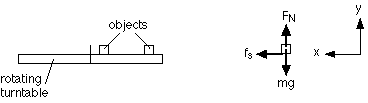Summing forces in the y-direction tells us that the normal force is equal in magnitude to the weight. In the x-direction, the only force there is is the frictional force.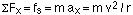The maximum possible value of the static force of friction is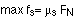As the velocity increases, the frictional force has to increase to provide the necessary force required to keep the object spinning in a circle. If we continue to increase the rotation rate of the turntable, thereby increasing the speed of an object sitting on it, at some point the frictional force won't be large enough to keep the object traveling in a circle, and the object will move towards the outside of the turntable and fall off.

Why does this happen to the outer objects first? Because the speed they're going is proportional to the radius (v = circumference / period), so the frictional force necessary to keep an object spinning on the turntable ends up also being proportional to the radius. More force is needed for the outer objects at a given rotation rate, and they'll reach the maximum frictional force limit before the inner objects will.